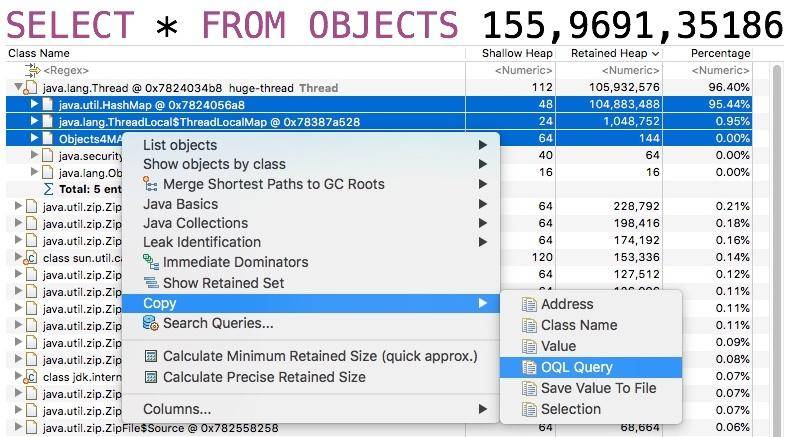13 工具进阶：如何利用 MAT 找到问题发生的根本原因

jmap 命令在 Java 9 之后，使用 jhsdb 命令替代，它们在用法上，区别不大。注意，这些命令本身会占用操作系统的资源，在某些情况下会造成服务响应缓慢，所以不要频繁执行。

``````jmap -dump:format=b,file=heap.bin 37340
jhsdb jmap  --binaryheap --pid  37340
``````

## 1. 工具介绍

MAT 工具是基于 Eclipse 平台开发的，本身是一个 Java 程序，所以如果你的堆快照比较大的话，则需要一台内存比较大的分析机器，并给 MAT 本身加大初始内存，这个可以修改安装目录中的 MemoryAnalyzer.ini 文件。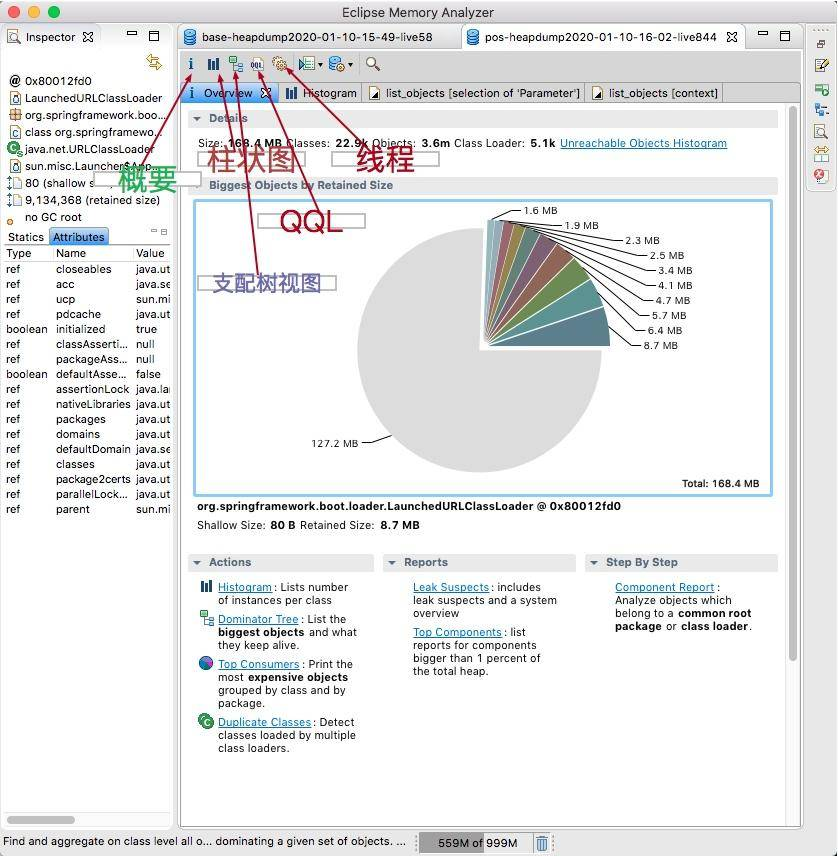• outgoing references 对象的引出
• incoming references 对象的引入

path to GC Roots 这是快速分析的一个常用功能，显示和 GC Roots 之间的路径。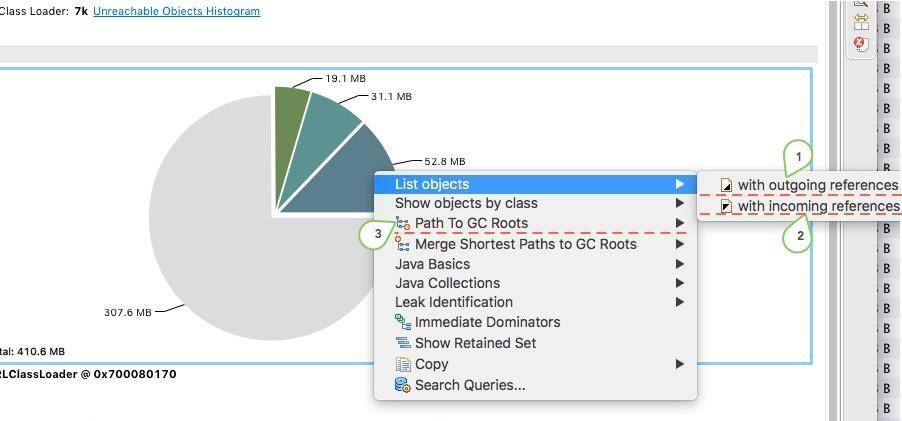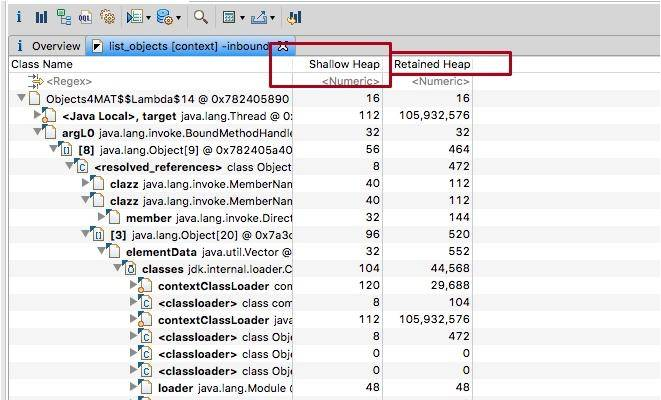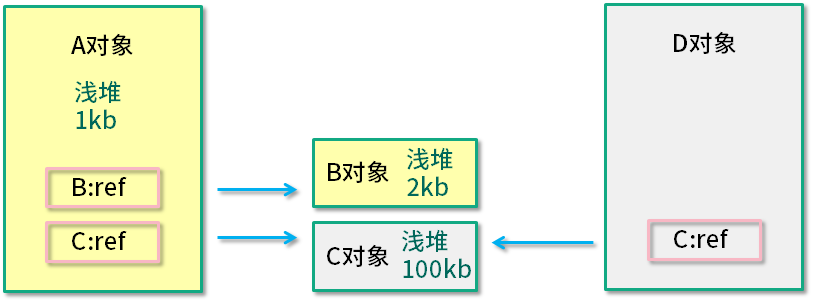A 对象大小（1 KB + 2 KB + 100 KB）> A 对象深堆 > A 对象浅堆。

## 2. 代码示例

``````import java.util.ArrayList;
import java.util.HashMap;
import java.util.List;
import java.util.Map;
import java.util.stream.IntStream;

public class Objects4MAT {

static class A4MAT {
B4MAT b4MAT = new B4MAT();
}

static class B4MAT {
C4MAT c4MAT = new C4MAT();
}

static class C4MAT {
List<String> list = new ArrayList<>();
}

static class DominatorTreeDemo1 {
DominatorTreeDemo2 dominatorTreeDemo2;

public void setValue(DominatorTreeDemo2 value) {
this.dominatorTreeDemo2 = value;
}
}

static class DominatorTreeDemo2 {
DominatorTreeDemo1 dominatorTreeDemo1;

public void setValue(DominatorTreeDemo1 value) {
this.dominatorTreeDemo1 = value;
}
}

static class Holder {
DominatorTreeDemo1 demo1 = new DominatorTreeDemo1();
DominatorTreeDemo2 demo2 = new DominatorTreeDemo2();

Holder() {
demo1.setValue(demo2);
demo2.setValue(demo1);
}

private boolean aBoolean = false;
private char aChar = '\0';
private short aShort = 1;
private int anInt = 1;
private long aLong = 1L;
private float aFloat = 1.0F;
private int[] ints = new int;
private String string = "1234";
}

Runnable runnable = () -> {
Map<String, A4MAT> map = new HashMap<>();

IntStream.range(0, 100).forEach(i -> {
byte[] bytes = new byte[1024 * 1024];
String str = new String(bytes).replace('\0', (char) i);
A4MAT a4MAT = new A4MAT();

map.put(i + "", a4MAT);
});

Holder holder = new Holder();

try {
//sleep forever , retain the memory
} catch (InterruptedException e) {
e.printStackTrace();
}
};

}

public static void main(String[] args) throws Exception {
Objects4MAT objects4MAT = new Objects4MAT();
}
}
``````

### 2.1. 代码介绍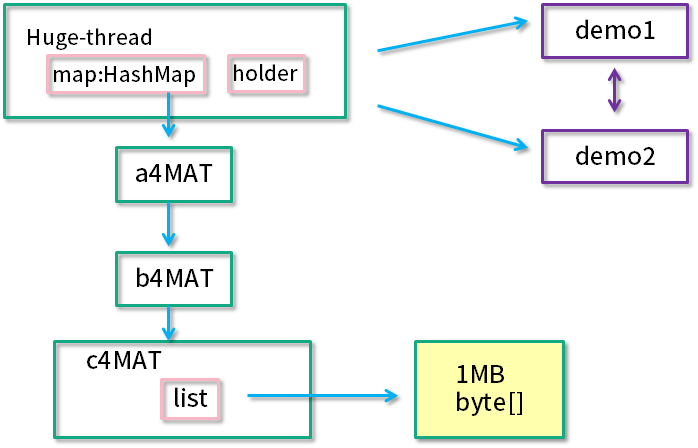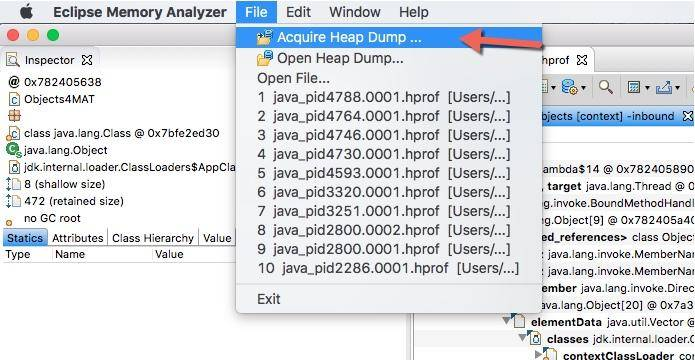### 2.2. 内存泄漏检测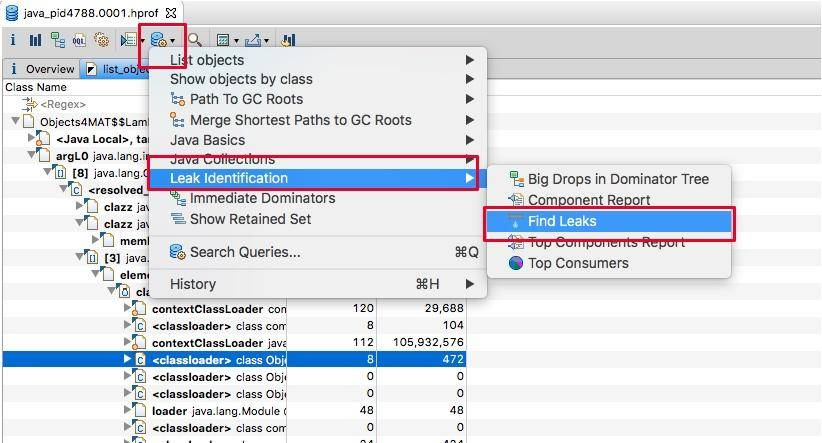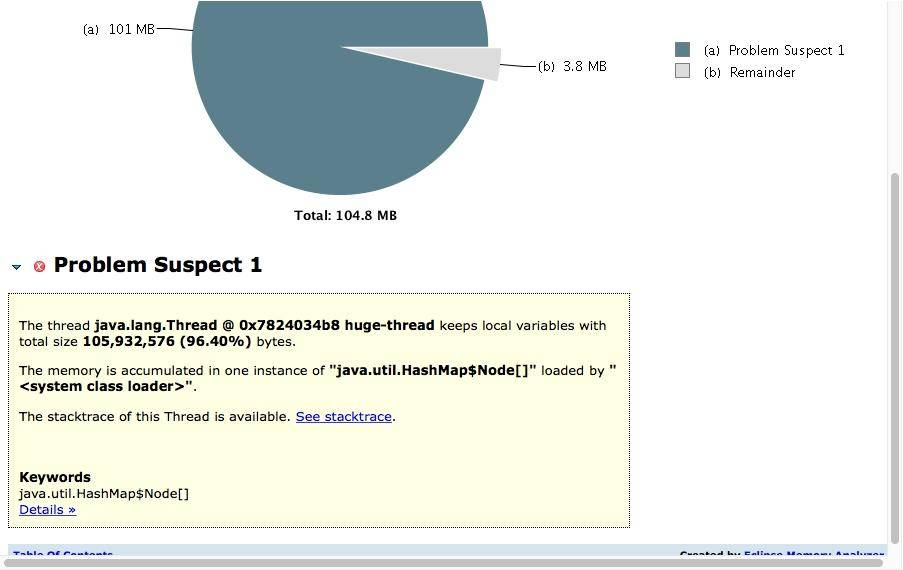### 2.3. 支配树视图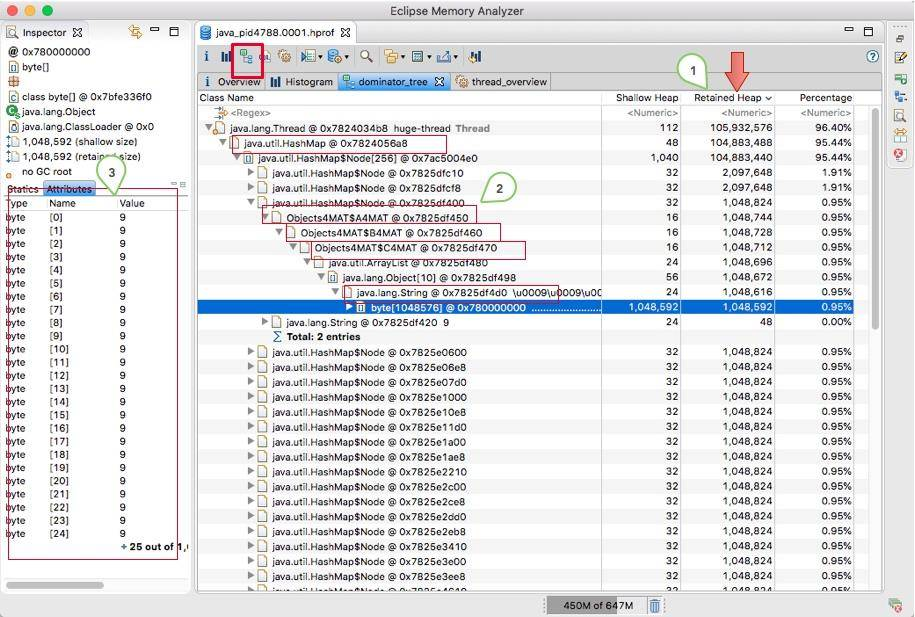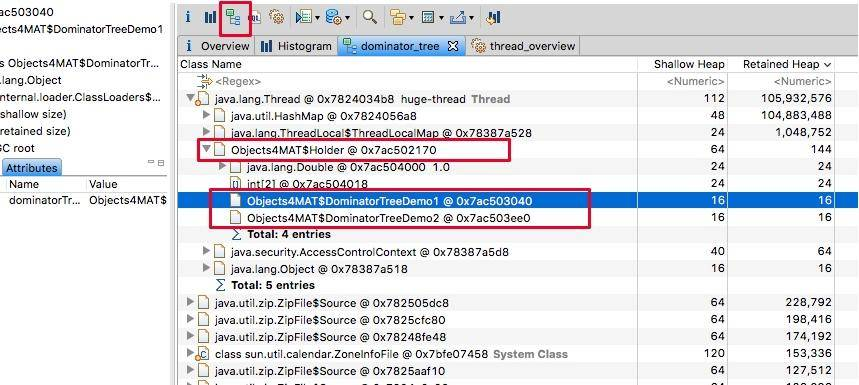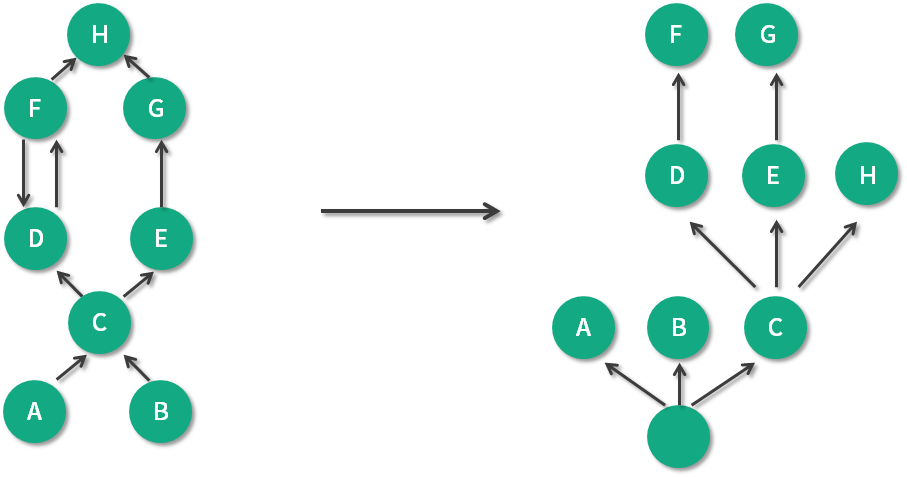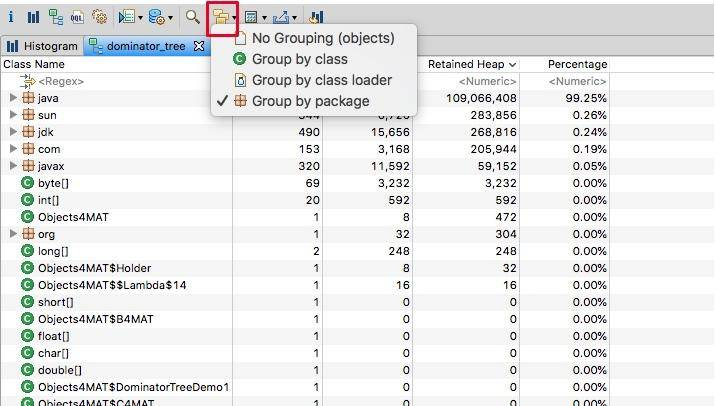``````public class DorminatorTreeDemo {
static class A {
C c;

byte[] data = new byte[1024 * 1024 * 2];
}

static class B {
C c;
byte[] data = new byte[1024 * 1024 * 3];
}

static class C {
D d;
E e;
byte[] data = new byte[1024 * 1024 * 5];
}

static class D {
F f;
byte[] data = new byte[1024 * 1024 * 7];
}

static class E {
G g;
byte[] data = new byte[1024 * 1024 * 11];
}

static class F {
D d;
H h;
byte[] data = new byte[1024 * 1024 * 13];
}

static class G {
H h;
byte[] data = new byte[1024 * 1024 * 17];
}

static class H {
byte[] data = new byte[1024 * 1024 * 19];
}

A makeRef(A a, B b) {
C c = new C();
D d = new D();
E e = new E();
F f = new F();
G g = new G();
H h = new H();
a.c = c;
b.c = c;
c.e = e;
c.d = d;
d.f = f;
e.g = g;
f.d = d;
f.h = h;
g.h = h;
return a;
}

static A a = new A();
static B b = new B();

public static void main(String[] args) throws Exception {

new DorminatorTreeDemo().makeRef(a, b);

}
}
``````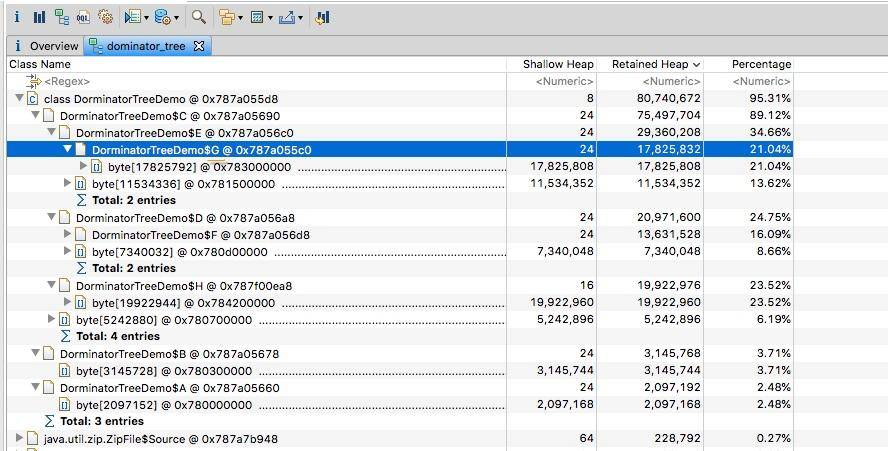### 2.5. 柱状图视图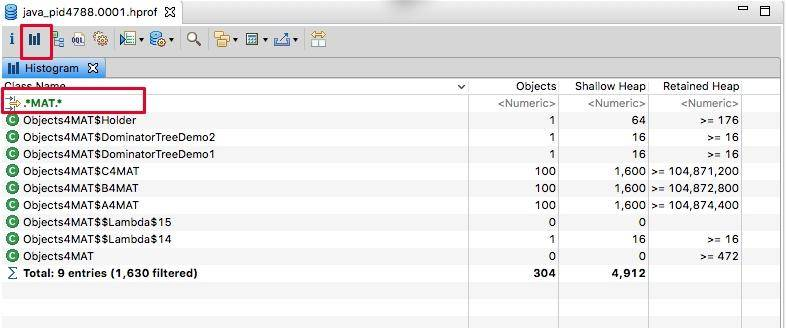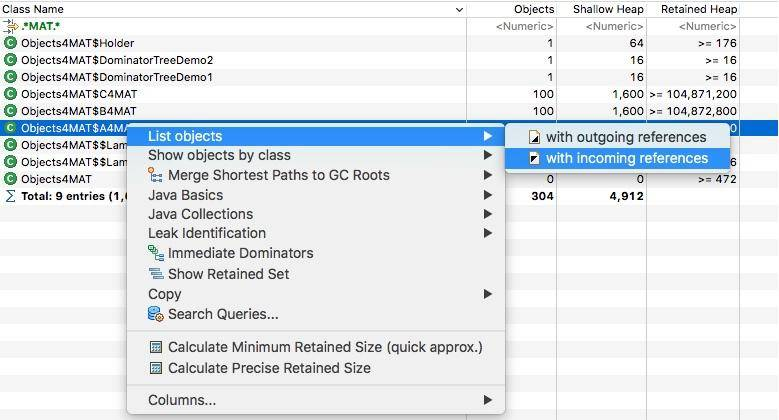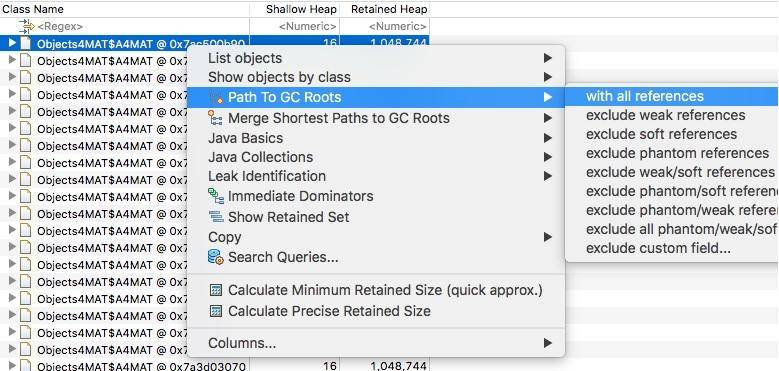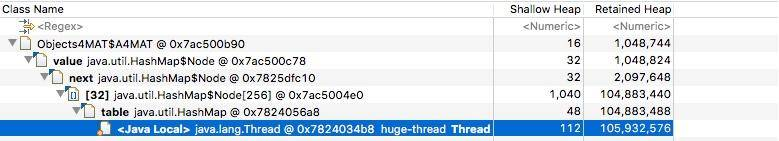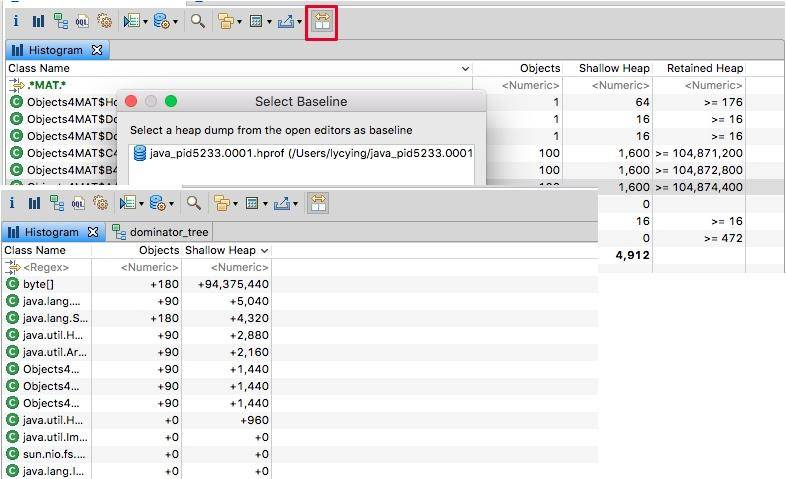## 3. 高级功能—OQL

MAT 支持一种类似于 SQL 的查询语言 OQL（Object Query Language），这个查询语言 VisualVM 工具也支持。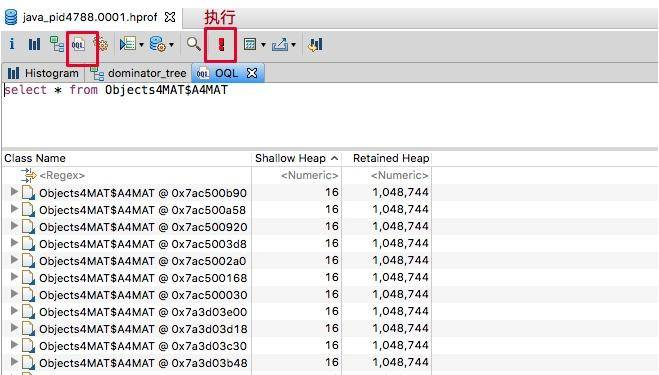``````SELECT * FROM  Objects4MAT\$A4MAT
``````

``````SELECT * FROM ".*MAT"
``````

``````SELECT OBJECTS s.value FROM java.lang.String s
SELECT OBJECTS mat.b4MAT FROM  Objects4MAT\$A4MAT mat
``````

``````select * from 0x55a034c8
``````

``````SELECT * FROM INSTANCEOF java.util.AbstractCollection
``````

``````SELECT * FROM byte[] s WHERE s.@length>1000
``````

``````SELECT * FROM java.lang.String s WHERE toString(s) LIKE ".*java.*"
``````

``````SELECT * FROM INSTANCEOF java.lang.Object o WHERE o.@retainedHeapSize>10000
``````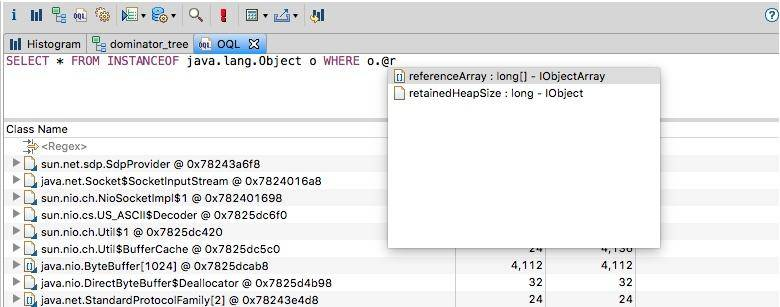OQL 有比较多的语法和用法，若想深入了解，可参考这里

OQL 还有一个好处，就是可以分享。如果你和同事同时在分析一个大堆，不用告诉他先点哪一步、再点哪一步，共享给他一个 OQL 语句就可以了。